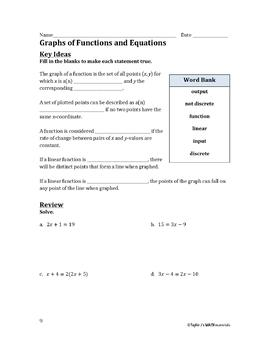# Graphs of Functions and Equations WorksheetSubject
Resource Type
File Type

PDF

(10 MB|4 pages)
Product Rating
Standards
Also included in:
1. Are you working on a unit on functions? This bundle is PACKED with problems that help students examine linear and nonlinear functions as equations, tables and graphs. They will also describe the functions and touch on discrete and not discrete functions!** SAVE 20% when you purchase the Complete Pa
\$6.25
\$5.50
Save \$0.75
• Product Description
• StandardsNEW

What is covered:

- Students review the meaning functions, linear functions, discrete and not discrete

- Students review solving multi-step equations (in order to identify a linear function in slope-intercept form)

- Students create tables based on equations, graph the points, and answer questions about linear functions

- Students identify functions based on graphs

3 Student Pages; 3 Teacher Pages

Possible uses:

bellringer or warm up, exit ticket, homework, informal assessment or quiz, test prep

*************************************************************************************************************

You might be interested in:

- these FREEBIES

- the unit on Linear Equations

- the unit on Percents & Proportional Relationships

- the unit on Congruence

or my store for other material to supplement the 7th & 8th grade curriculum!

**If you have any requests and/or updates for my work, please message me!

Interpret the equation 𝘺 = 𝘮𝘹 + 𝘣 as defining a linear function, whose graph is a straight line; give examples of functions that are not linear. For example, the function 𝘈 = 𝑠² giving the area of a square as a function of its side length is not linear because its graph contains the points (1,1), (2,4) and (3,9), which are not on a straight line.
Compare properties of two functions each represented in a different way (algebraically, graphically, numerically in tables, or by verbal descriptions). For example, given a linear function represented by a table of values and a linear function represented by an algebraic expression, determine which function has the greater rate of change.
Understand that a function is a rule that assigns to each input exactly one output. The graph of a function is the set of ordered pairs consisting of an input and the corresponding output.
Total Pages
4 pages
Included
Teaching Duration
N/A
Report this Resource to TpT
Reported resources will be reviewed by our team. Report this resource to let us know if this resource violates TpT’s content guidelines.